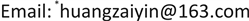1. 引言

2. 实验部分2.1. 试剂与仪器

2.2. 制备纳米CuS

2.3. 制备块体CuS

2.4. 电导率的测定

3. 结果与讨论

3.1. 溶解热力学函数

CuS ⇌ Cu 2 + + S 2 −

k s p = c ( Cu 2 + ) ⋅ c ( S 2 − ) = c 2 (1)

Λ m = k / c (2)

c为饱和溶液中微溶盐的溶解度，即：

Λ m ( 盐 ) = k ( 盐 ) / c (3)

Λ m ∞ ( CuS ) = Λ m ∞ ( Cu 2+ ) + Λ m ∞ ( S 2 − ) (4)

Λ m ∞ ( Cu 2 + ) = 5.32 × 10 − 3 ( S ⋅ m 2 ⋅ mol − 1 ) × 2 = 1 0. 64 × 1 0 − 3 ( S ⋅ m 2 ⋅ mol − 1 )

Λ m ∞ ( S 2 − ) = 51.2 × 10 − 3 ( S ⋅ m 2 ⋅ mol − 1 ) × 2 = 1 0 2 . 41 0 − 3 ( S ⋅ m 2 ⋅ mol − 1 )

Λ m ∞ ( CuS ) = 113.04 × 1 0 − 3 ( S ⋅ m 2 ⋅ mol − 1 )

Λ m ( CuS ) = Λ m ∞ ( CuS ) = 113.04 × 10 − 3 ( S ⋅ m 2 ⋅ mol − 1 )

c S 2 − = c CuS

c CuS = k CuS Λ m ( CuS ) (4)

k (溶液) = k (水) + k (盐)，k (盐) = k (溶液) − k (水)，式中k (盐)是纯微溶盐的电导率。

C = K CuS Λ m CuS

K s p = C 2

Δ G m θ = − R T ln K θ

Conductivity of pure water and copper sulfide solution at different temperature

100.77410.5536.7
0.74310.3336.2
0.71410.3136.2

201.04712.9646.2
0.95113.0246.2
0.95412.9446.2

301.09915.7256.5
1.11215.756.9
1.10915.756.9

401.24918.4567.7
1.2118.4768.3
1.20618.4768.3

501.32221.380.2
1.35821.480.3
1.39021.580.2

Conductivity of copper sulfide at different temperature

288.159.62135.591
298.1511.96545.192
308.1514.56455.624
318.1517.20266.839
328.1520.04378.876

Concentration of copper sulfide at different temperature

288.150.00850.0315
298.150.01060.0400
308.150.01290.0492
318.150.01520.0591
328.150.01770.0698

Δ G m θ = − R T ln K θ

Δ G m θ = Δ H m θ − T Δ S m θ

3.2. 表面热力学函数

Dissolution equilibrium constants of copper sulfide at the same temperatur

288.157.24 × 10−59.91 × 10−4
298.151.12 × 10−41.60 × 10−3
308.151.66 × 10−42.42 × 10−3
318.152.32 × 10−43.50 × 10−3
328.153.14 × 10−44.87 × 10−3

Gibbs free energy of copper sulfide at different temperature

288.1522.8416.57
298.1522.5515.96
308.1522.3015.43
318.1522.1414.96
328.1522.0014.53

Nano-CuS surface thermodynamic function
H m , CuS ( nano ) s Θ ( kJ ⋅ mol − 1 )G m , CuS ( nano ) s Θ ( kJ ⋅ mol − 1 )S m , CuS ( nano ) s Θ ( J ⋅ mol − 1 ⋅ K − 1 )
298.15 K2.33−6.5929.9

4. 结论